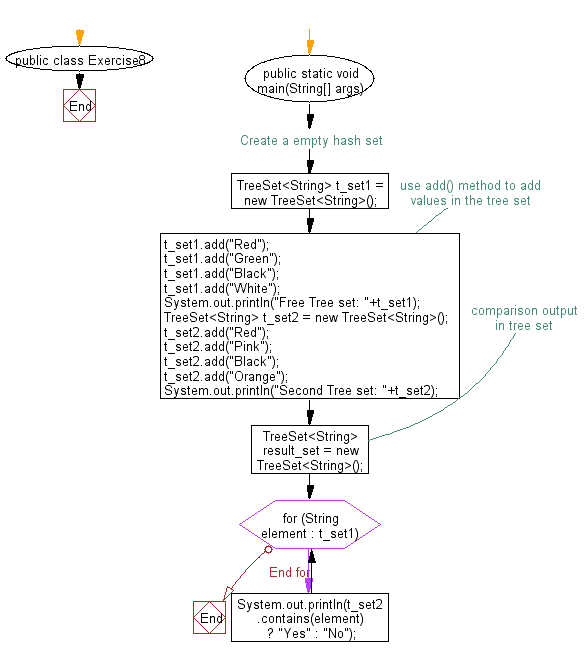﻿ Java - Compare two tree sets

# Java: Compare two tree sets

## Java Collection, TreeSet Exercises: Exercise-8 with Solution

Write a Java program to compare two tree sets.

Sample Solution:

Java Code:

``````import java.util.TreeSet;
import java.util.Iterator;

public class Exercise8 {
public static void main(String[] args) {
// Create a empty tree set
TreeSet<String> t_set1 = new TreeSet<String>();
System.out.println("Free Tree set: "+t_set1);

TreeSet<String> t_set2 = new TreeSet<String>();
System.out.println("Second Tree set: "+t_set2);
//comparison output in tree set
TreeSet<String> result_set = new TreeSet<String>();
for (String element : t_set1){
System.out.println(t_set2.contains(element) ? "Yes" : "No");
}
}
}
``````

Sample Output:

```Free Tree set: [Black, Green, Red, White]
Second Tree set: [Black, Orange, Pink, Red]
Yes
No
Yes
No ```

Flowchart:Java Code Editor:

What is the difficulty level of this exercise?

Test your Programming skills with w3resource's quiz.

﻿

## Java: Tips of the Day

Oddness Check

Is it possible to use this code to accurately determine the odd number?

```public boolean oddOrNot(int num) {
return num % 2 == 1;
}
```

I hope you noticed a trick. If we decide to check a negative odd number in such a way (-5, for example), the remainder of the division will not be equal to one (what does it mean?) Therefore, use a more accurate method:

```public boolean oddOrNot(int num) {
return (num & 1) != 0;
}
```

It not only solves the problem of negative numbers, but also works more productively than its predecessor. Arithmetic and logical operations are performed much faster than multiplication and division.

Ref: https://bit.ly/3giyonA

We are closing our Disqus commenting system for some maintenanace issues. You may write to us at reach[at]yahoo[dot]com or visit us at Facebook